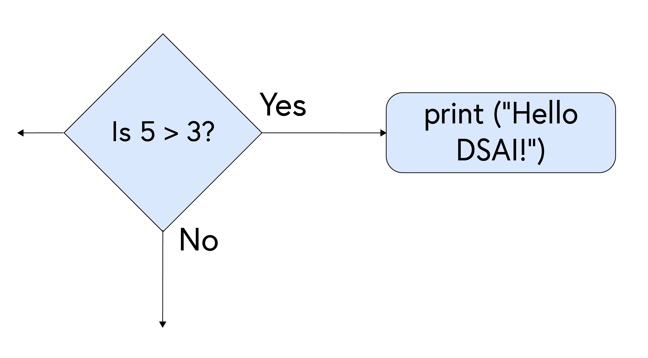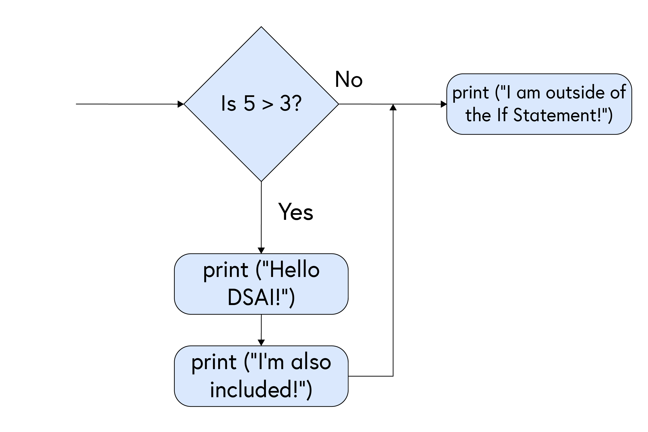## Want to keep learning?

This content is taken from the Coventry University's online course, Get ready for a Masters in Data Science and AI. Join the course to learn more.
1.22

# If, Elif and Else statements

In the previous step we looked at what conditional expressions are, but how do we use them in practice? This is where the If statement comes in.

We’ve actually seen an example of an If statement earlier in the course when we looked at flowcharts. They’re the decision blocks (the diamond-shaped ones) - does the user want milk, yes or no?

Let’s move on from tea and coffee and reconsider the opening example of the previous step on conditional expressions: “Is 5 Greater than 3?” Depending on the answer the user selects (yes or no), what do we want the program to do next? In this case, if the user selects ‘yes’, our program will continue down the Yes branch and will run print("Hello DSAI!").Before we launch into how to write the above example in Python, let’s take a look at the syntax for the If Statement in Python first.

### Syntax

There are two components of an If statement: the If keyword and the ConditionalExpression.

The If keyword should be the first thing you write, before the conditional expression. It is written simply as:

if():


The conditional expression is written between the brackets of the If keyword. This is where we ask our question, the answer to which will be either True or False. If the ConditionalExpression is True, the code in the IndentedStatementBlock will be run. If it’s False, however, then the indented code will be skipped.

if (ConditionalExpression):
IndentedStatementBlock


Returning to our example ‘Is 5 Greater than 3?’, if we wanted to turn it into an If statement we would simplify the question into a conditional expression:

if(5 > 3):


Then, any code we want to execute as a result of our conditional expression being true, we would insert below the expression, indented by four spaces.

if(5 > 3):
print("Hello DSAI!")


We use the indentation in Python to show the flow of the program. As long as we keep our code indented, we can add more lines to our If statement. To exit the If statement, you simply remove the indentation as shown below.

if(5 > 3):
print("Hello DSAI!")
print("I'm also included!")

print("I am outside of the If Statement!")


As a flowchart, this would now look like:### The Elif statement

We can use the Elif (Else If) statement to check for more conditions, but only if the previous conditions were not true. Take this flowchart example:

![Flowchart showing: Is X greater than Y? [diamond] - Yes - print (“X is greater than Y”) [rectangle]. No - Is X less than Y? [diamond] - Yes - print (“X is less than Y”) [rectangle]. No, continue. (Ehttps://ugc.futurelearn.com/uploads/assets/9d/1b/9d1b8934-1a8c-4367-9eec-98a09a26152e.png)

We could convert this flowchart to a program quite easily:

X = 15
Y = 10

if(X > Y):
print("X is Greater than Y")
if(X < Y):
print("X is Less than Y")


In this case, our first If statement will resolve to True, as X is Greater than Y. This means, continuing onto the next If statement would be a waste of processing power because we’ve already decided that X is Greater than Y. To avoid this, we use the Elif statement, causing the previous If statement to be skipped if resolved as True.

X = 15
Y = 10

if(X > Y):
print("X is Greater than Y")
elif(X < Y): #I will be skipped since the If statement above me was True
print("X is Less than Y")


### The Else statement

The Else statement is a very handy function as well. We can use it to catch anything that isn’t caught by the previous conditions, eg height or age restriction. If used, it must always be at the bottom of your If/Elifs.

X = 15
Y = 10

if(X > Y):
print("X is Greater than Y")
elif(X < Y): #I will be skipped since the If statement above me was True
print("X is Less than Y")
else:
print("X must be Equal to Y")


By using these conditional statements, we can start to gain more control over what our program executes and when it executes. We are moving beyond simple line-by-line execution. To continue practicing conditional statements, try experimenting with different operators in the task below.